Algebra 1 : How to find simple interest

Example Questions

1 3 Next →

Example Question #63 : Monetary Percentage

You deposit $2000 in a bank that earns 12% interest every year. How much interest will accrue in 5 years? Possible Answers: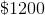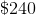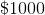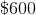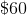Correct answer:Explanation: To find the interest earned, we use the following formula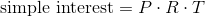where P is the principal or amount deposited, R is the rate of interest earned, and T is the time in years. Using this formula, we can substitute. We get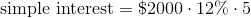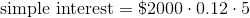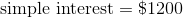Therefore, the amount of interested earned after 5 years is$1200.

You deposit $1500 into a savings account. The account earns 7% in interest annualy. How much simple interest will you earn in 6 years? Possible Answers: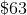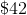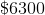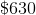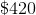Correct answer:Explanation: To find simple interest, we use the following formula:where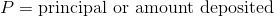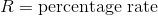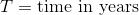Given what we know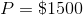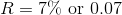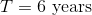we can substitute into the formula. We get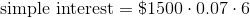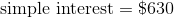Therefore, you earned a total ofin simple interest. Example Question #65 : Monetary Percentage You deposit$400 into a savings account.  The account earns 3% interest per year.  How much simple interest will you earn after 4 years?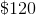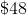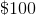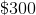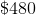Explanation:

To find simple interest, we use the following formula:whereGiven what we know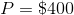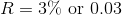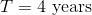we can substitute into the formula.  We get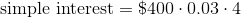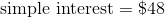Therefore, you earned a total ofin simple interest.

You invest $550 in a savings account that accrues interest at a rate of 6% annually. How much interest will you earn after 3 years? Possible Answers: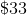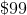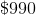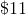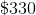Correct answer:Explanation: To find simple interest, we use the following formula:whereGiven what we know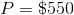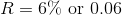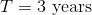we can substitute into the formula. We get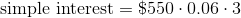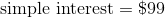Therefore, you earned a total ofin simple interest. Example Question #67 : Monetary Percentage You deposit$700 in a savings account at a bank.  It accrues 4% interest annually.  How much simple interest will you earn after 2.5 years?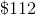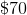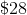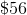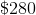Explanation:

To find simple interest, we use the following formula:whereGiven what we know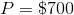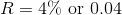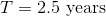we can substitute into the formula.  We get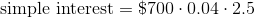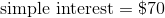Therefore, you earned a total ofin simple interest.

Example Question #68 : Monetary Percentage

Joey received a savings bond that pays out 4% interest each year. The face value of the bond is \$500. How much will Joey receive after holding the bond for one year?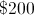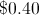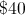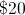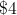Explanation:

Convert 4% into a decimal...

4% = 0.04

...and multiply by the face value of the bond. Ifis the interest paid after one year, the equation would look like this: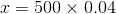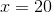1 3 Next →